Tamilnadu State Board New Syllabus Samacheer Kalvi 12th Maths Guide Pdf Chapter 10 Ordinary Differential Equations Ex 10.5 Textbook Questions and Answers, Notes.

## Tamilnadu Samacheer Kalvi 12th Maths Solutions Chapter 10 Ordinary Differential Equations Ex 10.5

Question 1.
If F is the constant force generated by the motor of an automobile of mass M, its velocity V is given by M $$\frac { dV }{ dt }$$ = F – kV, where k is a constant. Express V in terms of t given that V = 0 when t = 0.
Solution:
Given differential equation is M $$\frac { dV }{ dt }$$ = F – kV
The given equation can be written as
$$\frac { dV }{ F-kV }$$ = $$\frac { dt }{ M }$$
Now Integrating, we get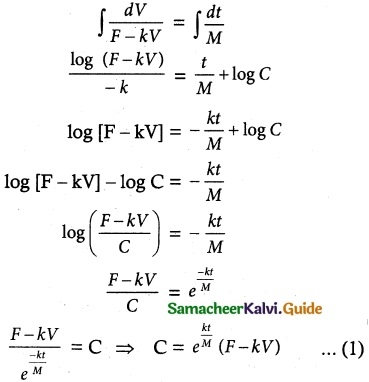Initial condition:
Given V = 0 When t = 0
C = e$$\frac { k(0) }{ M }$$ [F – k (0)]
= e° [F – 0]
C = F
∴ (1) ⇒ F = (F – kV)e$$\frac { kt }{ M }$$Question 2.
The velocity v, of a parachute falling vertically satisfies the equation v$$\frac { dv }{ dx }$$ = g(1 – $$\frac { v^2 }{ k^2 }$$) where g and k are constants. If v and are both initially zero, find v in terms of x.
Solution: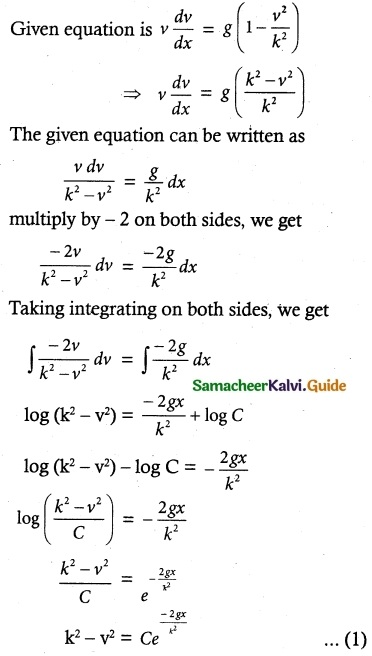Initial condition:
Given V = 0 when x = 0, we get
k²(0)² = Ce$$\frac { -2g(0) }{ k^2 }$$
k² = Ce°
k² = C
(1) ⇒ k² – v² = k² e$$\frac { -2gx }{ k^2 }$$
k² – k² e$$\frac { -2gx }{ k^2 }$$ = v²
k² [1 – e$$\frac { -2gx }{ k^2 }$$] = v²Question 3.
Find the equation of the curve whose slope is $$\frac { y-1 }{ x^2+x }$$ and which passes through the point (1, 0).
Solution: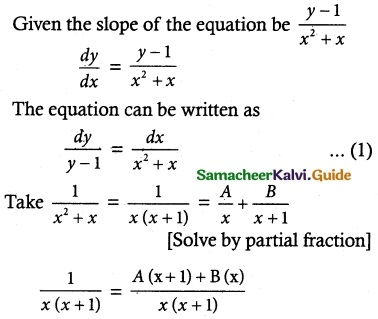1 = A(x + 1) + B(x)
Put x = -1; Put x = 0
1 = A (0) + B (-1) ; 1 = A(0 + 1) + B(0)
1 = -B; 1 = A
B = -1; A = 1
∴ $$\frac { 1 }{ x^2+x }$$ = $$\frac { 1 }{ x }$$ + $$\frac { 1 }{ x+1 }$$ ……. (2)
Substituting equation (2) in equation (1), we get
$$\frac { dy }{ y-1 }$$ = $$\frac { dx}{ x }$$ + $$\frac { dx }{ x+1 }$$
Taking integrating on both sides, we get
log (y – 1) = log x – log (x + 1) + log C
log (y – 1) = log C + log x – log (x + 1)
= log Cx – log (x + 1)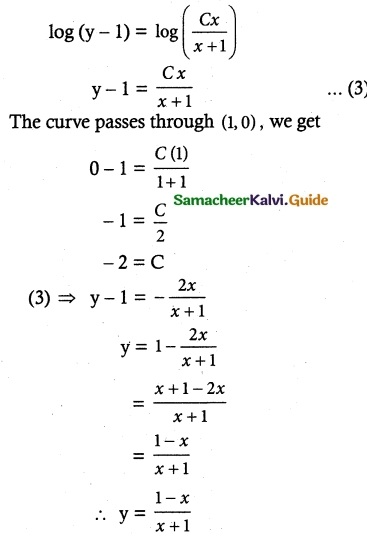Question 4.
Solve the following differential equations:
(i) $$\frac { dy }{ dx }$$ = $$\sqrt { 1-y^2 }{ 1-x^2 }$$
(ii) ydx + (1 + x²) tan-1 x dy = 0
(iii) sin $$\frac { dy }{ dx }$$ = a, y (0) = 1
(iv) $$\frac { dy }{ dx }$$ex+y + x³, ey
(v) (ey + 1) cos x dx + ey sin x dy = 0
(vi) (ydx – xdy) cot ($$\frac { x }{ y }$$) = ny² dx
(vii) $$\frac { dy }{ dx }$$ – x$$\sqrt { 25-x^2 }$$ = 0
(viii) x cos y dy = ex (x log x + 1) dx
(xi) tan y $$\frac { dy }{ dx }$$ = cos (x + y) + cos (x – y)
(x) $$\frac { dy }{ dx }$$ = tan² (x + y)
Solution:
(i) $$\frac { dy }{ dx }$$ = $$\sqrt { 1-y^2 }{ 1-x^2 }$$
The equation can be written as
$$\frac { dy }{ \sqrt {1-y^2} }$$ = $$\frac { dx }{ \sqrt {1-x^2} }$$
Taking Integration on both sides, we get
∫ $$\frac { dy }{ \sqrt {1-y^2} }$$ = ∫ $$\frac { dx }{ \sqrt {1-x^2} }$$
sin-1y = sin-1 x + C(ii) ydx + (1 + x²) tan-1 x dy = 0
ydx = – (1 + x²) tan-1 x dy
Take t = tan-1 x
dt = $$\frac { 1 }{ 1+x^2 }$$ dx
The equation can be written as
$$\frac { dx }{ (1+x^2)tan^{-1}x }$$ = –$$\frac { dy }{ y }$$
$$\frac { dt }{ t }$$ = –$$\frac { dy }{ y }$$
Taking Integration on both sides, we get
∫ $$\frac { dt }{ t }$$ = ∫ $$\frac { dy }{ y }$$
log t = – log y + log C
log (tan-1 x) = – log y + log C
log (y(tan-1 x)) + log y = log C
y tan-1 x = C

(iii) sin $$\frac { dy }{ dx }$$ = a, y (0) = 1
sin $$\frac { dy }{ dx }$$ = a
sin $$\frac { dy }{ dx }$$ = sin-1 (a)
The equation can be written as
dy = sin-1 (a) dx
Taking integration on both sides, we get
∫ dy = ∫ sin-1 (a) dx
y = sin-1 a ∫ dx
y = sin-1 (a) x + C ……… (1)
Initial condition:
Since y (0) = 1, we get
y = sin-1 (a) x + C
1 = sin-1 (a) (0) + C
0 + C = 1
C = 1
Equation (1) ⇒ y = sin-1 (a) x + 1
y – 1 = sin-1 (a) x
$$\frac { y-1 }{ x }$$ = sin-1 (a)
sin($$\frac { y-1 }{ x }$$) = a(iv) $$\frac { dy }{ dx }$$ex+y + x³, ey
$$\frac { dy }{ dx }$$ex+y + x³, (ey)
= ey [ex + x³]
$$\frac { dy }{ e^y }$$ = dx(ex + x³)
The equation can be written as
$$\frac { dy }{ e^y }$$ = (ex + x³) dx
Taking integration on both sides, we get
∫ e-y dy = ∫ (ex + x³) dx
$$\frac { e^y }{ -1 }$$ = ex + $$\frac { x^4 }{ 4 }$$ + C
[Where – C = C, Which is also constant].
∴ ex + e-y + $$\frac { x^4 }{4 }$$ = -C = C
∴ ex + e-y + $$\frac { x^4 }{4 }$$ = C

(v) (ey + 1) cos x dx + ey sin x dy = 0
Solution:
(ey + 1) cos x dx + ey sin x dy = 0
ey sin x dy = – (ey + 1) cos x dxlog (ey + 1) = – log sin x + log c
log [(ey + 1) + log sin x = log c
log (ey +1) sin x] = log c
(ey+ 1) sin x = c(vi) (ydx – xdy) cot ($$\frac { x }{ y }$$) = ny² dx
equation can be written asSubstituting these values in equation (1), we get
dt cot t = ndx
cot t dt = ndx
Taking integration on both sides, we get
∫ cot t dt = n∫ dx
log (sin t) = n x + c
sin t = enx+c
∴ sin($$\frac { x }{ y }$$) = enx+c [∵ t = $$\frac { x }{ y }$$ ]

(vii) $$\frac { dy }{ dx }$$ – x$$\sqrt { 25-x^2 }$$ = 0
The equation can be written as
$$\frac { dy }{ dx }$$ – x$$\sqrt { 25-x^2 }$$ …….. (1)
Take 25 – x² = t
-2x dx = dt
x dx = –$$\frac { dt }{ 2 }$$
Substituting these values in equation (1), we get
dy = x$$\sqrt { 25-x^2 }$$ dx
dy = -√t $$\frac { dt }{ 2 }$$
Taking integration on both sides, we get
∫ dy = –$$\frac { dt }{ 2 }$$ ∫ t$$\frac { 1 }{ 2 }$$ dt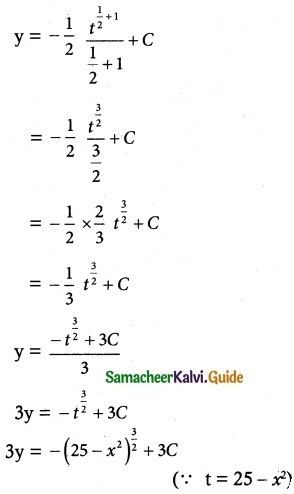(viii) x cos y dy = ex (x log x + 1) dx
The equation can be written asSubstituting in (1), we get
sin y = ey log x + C(ix) tan y $$\frac { dy }{ dx }$$ = cos (x + y) + cos (x – y)
The equation can be written as
tan y $$\frac { dy }{ dx }$$ = cos (x + y) + cos (x – y)
[W.K.T cos (A + B) + cos (A – B) = 2 cos A cos B
Here A = x, B = y]
∴ tan y$$\frac { dy }{ dx }$$ = 2 cos x cos y
$$\frac { tany }{ cosy }$$ dy = 2 cos x dx
Taking integration on both sides, we get
∫ $$\frac { tany }{ cosy }$$ dy = 2 ∫ cos x dx
2 ∫ tan y sec y dy = 2 ∫ cos x dx
sec y = 2 sin x + C

(x) $$\frac { dy }{ dx }$$ = tan² (x + y)